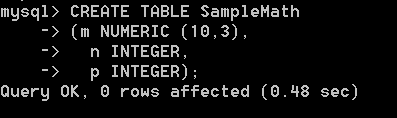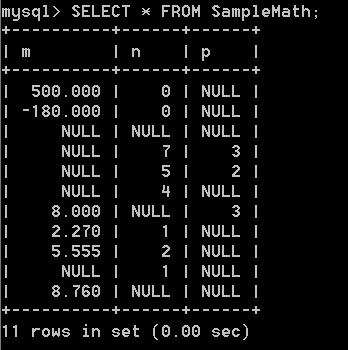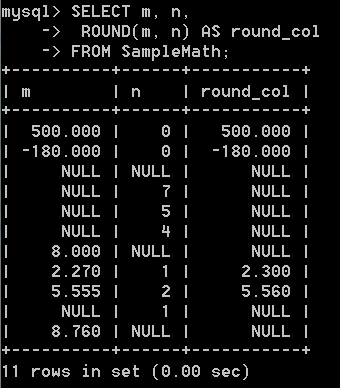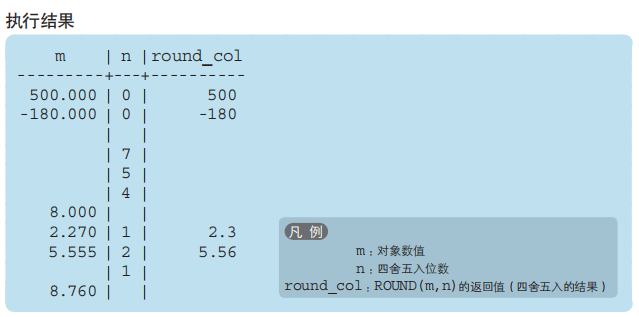2019-09-01 19:19

# MySQL 对NUMERIC类型数据使用 ROUND函数

``````-- DDL ：创建表
CREATE TABLE SampleMath
(m NUMERIC (10,3),
n INTEGER,
p INTEGER);

-- DML ：插入数据
START TRANSACTION;
INSERT INTO SampleMath(m, n, p) VALUES (500, 0, NULL);
INSERT INTO SampleMath(m, n, p) VALUES (-180, 0, NULL);
INSERT INTO SampleMath(m, n, p) VALUES (NULL, NULL, NULL);
INSERT INTO SampleMath(m, n, p) VALUES (NULL, 7, 3);
INSERT INTO SampleMath(m, n, p) VALUES (NULL, 5, 2);
INSERT INTO SampleMath(m, n, p) VALUES (NULL, 4, NULL);
INSERT INTO SampleMath(m, n, p) VALUES (8, NULL, 3);
INSERT INTO SampleMath(m, n, p) VALUES (2.27, 1, NULL);
INSERT INTO SampleMath(m, n, p) VALUES (5.555,2, NULL);
INSERT INTO SampleMath(m, n, p) VALUES (NULL, 1, NULL);
INSERT INTO SampleMath(m, n, p) VALUES (8.76, NULL, NULL);
COMMIT;
``````- 书里面给的查询示例却是这样的？这个是出了什么状况了？bug吗？还是版本的问题？ 我电脑装的是MySQL5.7.26版本。 . 书上的示例查询结果如下：• 点赞
• 写回答
• 关注问题
• 收藏
• 邀请回答